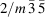International
Tables for
Crystallography
Volume A
Space-group symmetry
Edited by M. I. Aroyo

International Tables for Crystallography (2016). Vol. A, ch. 3.2, pp. 735-737

Section 3.2.1.4.3. Sub- and supergroups of the general point groups

Th. Hahna and H. Klappera

3.2.1.4.3. Sub- and supergroups of the general point groups

| top | pdf |

In Figs. 3.2.1.4to 3.2.1.6, the subgroup and supergroup relations between the two-dimensional and three-dimensional general point groups are illustrated. It should be remembered that the index of a group–subgroup relation between two groups of order infinity may be finite or infinite. For the two spherical groups, for instance, the index is ; the cylindrical groups, on the other hand, are subgroups of index [] of the spherical groups.Figure 3.2.1.4 | top | pdf |Subgroups and supergroups of the two-dimensional general point groups. Solid lines indicate maximal normal subgroups, double solid lines mean that there are two maximal normal subgroups with the same symbol. Dashed lines refer to sets of maximal conjugate subgroups. For the finite groups, the orders are given on the left. Note that the subgroups of the two circular groups are not maximal and the diagram applies only to a specified value of N (see text). For complete examples see Fig. 3.2.1.5.Figure 3.2.1.5 | top | pdf |The subgroups of the two-dimensional general point groups 16mm (4N-gonal system) and 18mm [-gonal system, including the-gonal groups]. Compare with Fig. 3.2.1.4which applies only to one value of N.Figure 3.2.1.6 | top | pdf |Subgroups and supergroups of the three-dimensional general point groups. Solid lines indicate maximal normal subgroups, double solid lines mean that there are two maximal normal subgroups with the same symbol. Dashed lines refer to sets of maximal conjugate subgroups. For the finite groups, the orders are given on the left and on the right. Note that the subgroups of the five cylindrical groups are not maximal and that the diagram applies only to a specified value of N (see text). Only those crystallographic point groups are included that are maximal subgroups of noncrystallographic point groups, cf. Fig. 3.2.1.3. Full Hermann–Mauguin symbols are used.

Fig. 3.2.1.4for two dimensions shows that the two circular groups ∞m and ∞ have subgroups of types nmm and n, respectively, each of index []. The order of the rotation point may beor. In the first case, the subgroups belong to the 4N-gonal system, in the latter two cases, they belong to the-gonal system. [In the diagram of the-gonal system, the-gonal groups appear with the symbolsand.] The subgroups of the circular groups are not maximal because for any given value of N there exists a group withwhich is both a subgroup of the circular group and a supergroup of the initial group.

The subgroup relations, for a specified value of N, within the 4N-gonal and the-gonal system, are shown in the lower part of the figure. They correspond to those of the crystallographic groups. A finite number of further maximal subgroups is obtained for lower values of N, until the crystallographic groups (Fig. 3.2.1.2) are reached. This is illustrated for both systems in Fig. 3.2.1.5.

Fig. 3.2.1.6for three dimensions illustrates that the two spherical groupsandeach have one infinite set of cylindrical maximal conjugate subgroups, as well as one infinite set of cubic and one infinite set of icosahedral maximal finite conjugate subgroups, all of index [].

Each of the two icosahedral groups 235 andhas one set of five cubic, one set of six pentagonal and one set of ten trigonal maximal conjugate subgroups of indices ,  and , respectively (cf. Section 3.2.1.4.2, The two icosahedral groups); they are listed on the right of Fig. 3.2.1.6. For the crystallographic groups, Fig. 3.2.1.3applies. The subgroup types of the five cylindrical point groups are shown on the upper left part of Fig. 3.2.1.6. As explained above for two dimensions, these subgroups are not maximal and of index []. Depending upon whether the main symmetry axis has the multiplicity 4N,or, the subgroups belong to the 4N-gonal,-gonal or-gonal system.

The subgroup and supergroup relations within these three systems are displayed in the lower left part of Fig. 3.2.1.6. They are analogous to the crystallographic groups. To facilitate the use of the diagrams, the-gonal and the-gonal systems are combined, with the consequence that the five classes of the-gonal system now appear with the symbolsand. Again, the diagrams apply to a specified value of N. A finite number of further maximal sub­groups is obtained for lower values of N, until the crystallographic groups (Fig. 3.2.1.3) are reached (cf. the two-dimensional examples in Fig. 3.2.1.5).